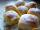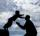# If-then equation

If 5x - 17 = -x + 7, then x =

Result

x =  4

#### Solution:

5x - 17 = -x + 7

6x = 24

x = 4

Calculated by our simple equation calculator.

Leave us a comment of example and its solution (i.e. if it is still somewhat unclear...):

Showing 1 comment:Math student
Word problems#### To solve this example are needed these knowledge from mathematics:

Do you have a linear equation or system of equations and looking for its solution? Or do you have quadratic equation?

## Next similar examples:

1. Temperature 2Sunday's high temperature was 3 degrees higher than Saturday's. On Monday, the temperature fell 5 degrees, then rose 7 degrees on Tuesday and 4 more on Wednesday. Then it fell 17 degrees to a record low of 31 on Thursday. What was the temperature on Saturd
2. Eq1Solve equation: 4(a-3)=3(2a-5)
3. Forest nurseryIn the forest nursery after winter, they found that 1/10 stems died out of them. For them, they land 193 new spruces. How many spruces are in the forest nursery?
4. CookiesMom bake cookies. Rolo took 2/9 of all cookies, Michal 3/9. How many cookies ate Rolo if Michal had 9.
5. Cakes1/3 poppy cake, 1/3 apple, 15 pieces of cheese. How many are totally cakes?
6. Jan and DanJan and Dan had the same money. Jan bought 5 workbooks and left him 15 CZK. Dan 6 and left him nothing. How much money have in total?
7. Apples 3Julka has 5 apples more than Hugo and four apples less than Annie. Hugo has 17 apples. How many apples has Julka and how Annie?
8. Jam cakesMom baked a third of plum jam cakes, one third cheesecakes and 18 poppy. How many cakes she had bake?
9. Father and sonFather is 44 years old, his son 16 years. Determine how many years ago was the father five times older than the son.
10. NumberDetermine unknown number if you know that difference between five times and triple of number is 42.
11. Seven timesWhich number seven times is just as higher as 27, how much is smaller than 29?
12. BenchesThe park has 64 benches. Occupied are by 18 more than empty. How many benches are occupied and empty ?
13. PopsiclesFrancis went to buy ice lollies. If he buy 8 popsicles he missed 4 USD. When he buy 7 popsicles, got back 1 USD. How many USD was a popsicle?
14. Number unknownAdela thought the two-digit number, she added it to its ten times and got 407. What number does she think?
15. Simple equation 5Solve equation with fractions: X × 3/8 = 1/2
16. Equation 20In given equation: 8/9-4/5=2/9+x, find x
17. Addition of Roman numbersAdded together and write as decimal number: LXVII + MLXIV